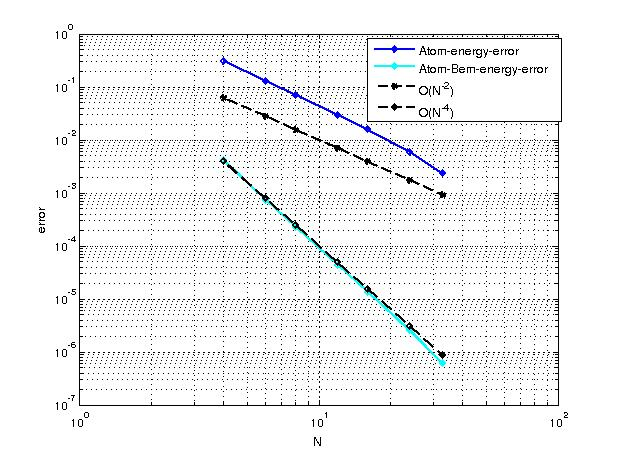# Atomistic / Linear Elasticity Coupling Model

## Coupling Methods

Assume that the whole space$\mathbb{R}^2 = \Omega^a \cup \Omega^l$ with interface$\Gamma := \Omega^a \cup \Omega^l$. It is obvious that$\min \mathcal{E}^{al}(u) = \min \mathcal{E}^r(u)$ where$\mathcal{E}^r(u) := \mathcal{E}^a(u) + \mathcal{E}^{\text{GRAC}}(u) + \min_{v|_{\Gamma} = I_hu_{\Gamma}} \mathcal{E}^l(v)$.

And minimising$\mathcal{E}^l(v)$ is equivalent to finding solution to the following exterior Laplacian problem$-\mu \Delta v = 0 \text{ in } \Omega^l$$v|_{\Gamma} = I_h u|_{\Gamma}$$v(x) \sim O\left(\frac{1}{|x|}\right) \text{ as } |x|\rightarrow \infty$

Since$v$ is defined in infinite domain, it is unrealistic to apply finite element method to solve the problem numerically, instead, one can use boundary integral method to solve it. Furthermore, the solution$v$ has the following integral representation in$\Omega^l$$v(x) = \int_{\Gamma} v(y)\frac{\partial G(x,y)}{\partial n (y)} - \frac{\partial v}{\partial n}(y) G(x,y) \text{d} s(y), \quad x\in \Omega^{l}$
with$G(x, y) := -\frac{1}{2\pi}\text{ln}(|x-y|)$ being the fundamental solution of Laplace equation in two dimensions. Using the Dirichlet boundary condition, we obtain$V\varphi = f \text{with unknown density} \varphi := \frac{\partial v}{\partial n} \text{and right hand side} f : =\left(K - \frac{1}{2} I\right) u$ where$(V\varphi)(x) := \int_{\Gamma}G(x, y)\varphi(y) \text{d} s(y) \quad (K\varphi)(x) := \int_{\Gamma}\frac{\partial G(x, y)}{\partial \nu (y)}\varphi(y) \text{d} s(y), \quad x \in \Gamma$

The integral equation has a unique solution in$H^{-\frac{1}{2}}_{\ast}(\Gamma) =\{\varphi \in H^{-\frac{1}{2}}(\Gamma): \int_{\Gamma}\varphi = 0\}$.

We will apply Galerkin boundary element method to discretize the integral equation. To that end, assume that$S_h^0(\Gamma) = \text{span}\{\varphi_k^0\}_{k=1}^M$ is piece-wise constant function space on$\Gamma$ and we define$S^0_{*, h}(\Gamma) := \{ \varphi_h(x)\in S^0_h(\Gamma): \varphi_h(x) = \sum_{k=1}^M a_k \varphi^0_k (x) \text{ with } \sum_{k=1}^M a_k = 0\}$

then we find solution$\varphi_h \in S^0_{*, h}(\Gamma)$ such that$\langle V\varphi_h, \psi_h \rangle = \langle f, \psi_h \rangle = \langle (K - \frac{1}{2}I)u, \psi_h \rangle, \text{ for } \psi_h\in S^0_{*, h}(\Gamma)$. And we obtain the approximate solution as follows$v_h(x) = \int_{\Gamma} u(y)\frac{\partial G(x,y)}{\partial n (y)} - \varphi_h(y) G(x,y) \text{d} s(y), \quad x\in \Omega^{l}$

We can transfer the boundary element discretisation error to the following consistency error of the linearisation energy$|\langle \delta \mathcal{E}^l(v), w \rangle - \langle \delta \mathcal{E}^l(v_h), w \rangle |\leq c N^{-\frac{5}{2}} h^{\frac{3}{2}} \|w\|_{H^{\frac{1}{2}}(\Gamma)}$

where$N$ denotes the radius of atomistic region. Recalling the consistency error for the GRAC energy in A/N/L coupling method is of$O(N^{-\frac{5}{2}})$, we obtain the consistency error for A/L coupling method$|\langle\delta \mathcal{E}^a(u) - \delta \mathcal{E}^{al}(u), w \rangle| \leq C N^{-\frac{5}{2}}|w|_{H^1}$
Under the assumption of stability, we obtain the following error estimate$|u^{a} - u^{al}|_{H^1} \leq CN^{-\frac{5}{2}}, \quad \mathcal{E}^{a}(u^a) - \mathcal{E}^{al}(u^{al}) \leq CN^{-5}$

## Numerical Results

The following figure shows the convergence rate for the error between atomistic energy and A/L energy, but the computational rate is$O(N^{-4})$ which seems contradicting with the theoretical rate. However, we are not clear whether the constant in the error estimate depends on$N$, so the theoretical rate might be$O(N^{-4})$ in that case.If we instead use the linearised Cauchy Born rule coupled to the atomistic region, and a FEM discretisation rather than a BEM one, we have the following results:As with the other tests we scaled the atomistic region using the power$3/2$ for practical reasons, and it is promising we again observe a higher rate than this.